An Existence Result for Impulsive Stochastic Functional Differential Equations with Multiple Delays | OMICS International
Journal of Physical Mathematics
All submissions of the EM system will be redirected to Online Manuscript Submission System. Authors are requested to submit articles directly to Online Manuscript Submission System of respective journal.

# An Existence Result for Impulsive Stochastic Functional Differential Equations with Multiple Delays

Anguraj A* and Banupriya K

P.S.G. College of Arts and Science, Coimbatore, Tamil Nadu, India

*Corresponding Author:
Anguraj A
P.S.G. College of Arts and Science
Tel: 0422 430 3300
E-mail: [email protected]

Received Date: April 11, 2015; Accepted Date: June 09, 2015; Published Date: June 16, 2015

Citation: Anguraj A, Banupriya K (2015) An Existence Result for Impulsive Stochastic Functional Differential Equations with Multiple Delays. J Phys Math 6:139. doi:10.4172/2090-0902.1000139

Copyright: © 2015 Anguraj A, et al. This is an open-access article distributed under the terms of the Creative Commons Attribution License, which permits unrestricted use, distribution, and reproduction in any medium, provided the original author and source are credited.

Visit for more related articles at Journal of Physical Mathematics

#### Abstract

In this paper we consider Impulsive stochastic neutral functional differential equations with multiple delays. By using Schaefer’s fixed point theorem, we prove the existence of solutions for stochastic differential equations with impulses

#### Keywords

Impulsive differential equations; Stochastic differential equations; Multiple delay; Fixed point theorem

AMS Mathematical Subject Classification: 34A37, 34K50, 34K45, 60H10.

#### Introduction

The theory of impulsive differential equations is an important area of scientific activity. Many evolution processes are characterized by the fact that at certain moments of time they experience a change of state abruptly. These short term perturbations act instantaneously, that is in the form of impulses. For example, that many biological phenomena involving thresholds, optimal control models in economics and frequency modulated systems, do exhibit impulsive effects. So the impulsive differential equations appear as a natural description of observed evolution phenomena of several real world problems. Existence of solutions of impulsive differential equations has been studied by many authors. If the impulses are random the solution becomes a stochastic process. Existence of solutions of differential equations with random impulses have been studied by many authors [1-3].

Furthermore, besides impulsive effects, stochastic effects likewise exist in real systems. There is a wide range of interesting process in robotics, economics and biology that can be described as differential equations with non-deterministic dynamics such phenomena are described by stochastic differential equations. The solution of stochastic differential equation is a stochastic process. However the solution of differential equation with random impulses is different from the solution of stochastic differential equations. Existence, Uniqueness and qualitative analysis of solutions of stochastic differential equations have discussed by several authors [4,5].

Since both impulsive and stochastic effects exist it is very difficult to investigate the existence of solution of impulsive stochastic differential equations. In  Anguraj and Vinodkumar discussed the existence, uniqueness and stability of impulsive stochastic semi linear neutral functional differential with infinite delays. Lakrib  discussed about the existence results for impulsive neutral functional differential equations with multiple delays. Based on the existing literature, stochastic impulsive differential equations involved mainly on controlability and stability. To the best of our knowledge, there is no work reported on impulsive stochastic differential equations with multiple delays. The purpose of this paper is to discuss about the existence results of impulsive stochastic neutral functional differential equations with multiple delays. Our approach is based on Schaefer’s fixed point theorem.

In this paper we study the existence results for stochastic impulsive differential equations with multiple delays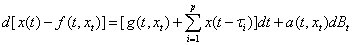(1.1)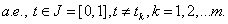Δx(tk )=Ik (x(tk)), k=1, 2, ...m

x0

where f : J × Rn → Rn, g : J × Rn → Rn and a : J × Rn → Rn are Borel Measurable functions, J0 =[−r, 0], r=max {τi : i=1, 2, ...p} and φ: [−r, 0] → Rn. Further-more the fixed moments of time tk satisfy 0=t0<t1<..... <tm<tm+1=1, where x(tk+) and x(tk-) represent the right and left limits of x(t) at t=tk, respectively. And Δx(t = tk) = x(tk+) - x(tk-) represent the jump in the state at time tk with Ik determining the size of the jump.

#### Preliminaries

Let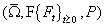be a complete probability space with a filtration {Ft}t≥0 satisfying the conditions that it is right continuous and F0 contains all P- null sets and w(t)=(w1(t), ....wm(t))T is an m-dimensional Brownian motion defined onLet C=C [[−r, 0], Rn] denote the family of all continuous Rn−valued function φ on [−r, 0] with the norm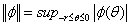where |.| is Euclidean norm of Rn. Denote bythe family of all bounded F0- measurable,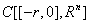-valued random variables φ, satisfying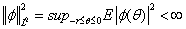, where E denotes the expectation of stochastic process [8-10]. The initial condition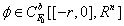.

Let PC(J, Rn) the space of piecewise continuous functions x: J →Rn such that x is continuous everywhere except for t=tk at which x(tk-) and x(tk+) exist and x(tk-) = x(tk) , k=1,...m. If we set Ω={x: J1 → Rn, x ∈ Rn ∩ PC (J, Rn)} where J1=[−r, 1] then Ω is a Banach space normed by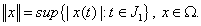.

Obviously, for any x∈ Ω and t ∈ J, the function xt defined by xt(θ)=x(t + θ), for θ ∈ J0, belongs to Rn.

By L1 (J, Rn) we denote the Banach space of measurable functions x: J → Rn which are Lebesgue integrable, normed by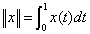Definition 2.1: A stochastic process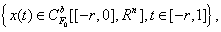is called a solution of equations (1.1) if

(ii)x(t) satisfies the integral equation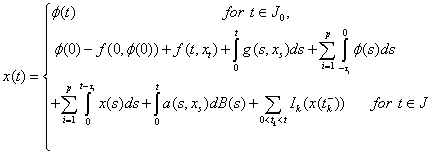(2.1)

Definition 2.2: (Schaefer fixed point theorem). Let X be a normed linear space and let Γ: X → X be a completely continuous map, that is, it is a continuous mapping which is compact on each bounded subset of X. If the set ζ={x ∈∈ X: λx=Γx for some λ>1} is bounded, then Γ has a fixed point .

#### Hypotheses

H1: The function f, g: J × Rn → Rn is such that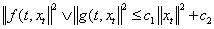for each t∈[0, 1] and c1, c2>0 are constants.

H2: The function a: J × Rn → Rn is Caratheodory, that is,

(i)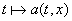is measurable for each x ∈ R,

(ii)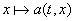is continuous for a.e t ∈ J.

H3: There exists a function q ∈ L1(J, Rn) with q(t)>0 for a.e t ∈ J and a continuous non decreasing function ψ : [0, ∞) → [0, ∞) such that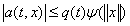for a.e t ∈ J and each x ∈ Rn with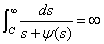(3.1)

Where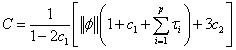H4: The function Ik: R → R and there exist positive constant ck, k=1, 2, ....m, such that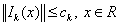Theorem 2.1: Suppose that the conditions (H1) − (H4) are satisfied then there exists a solution of the problem (1.1) on J1 [12-14].

Proof: Transform the problem (1.1) − (1.3) into a fixed point problem. Consider the operator Γ : Ω → Ω defined by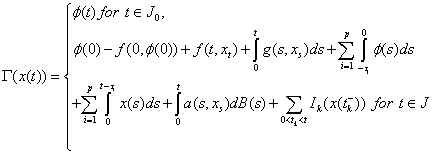Step 1: Γ has bounded values for bounded sets in Ω.

Let B be a bounded set in Ω. Then there exists a real number ρ>0 such that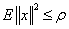, for all x ∈ B.

Let x ∈ B and t ∈ J, we have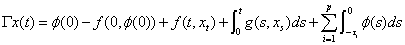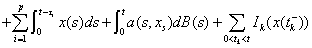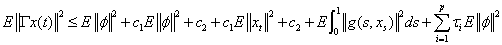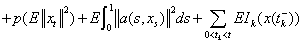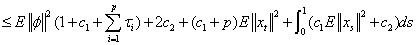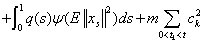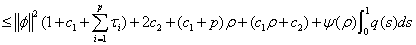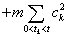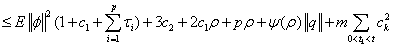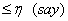If t ∈ J0, then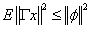and hence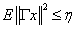for all x ∈ B, that is Γ is bounded on bounded subsets of Ω.

Step 2: Γ maps bounded sets into equicontinuous sets.

Let B be as in Step 1 and x ∈ B. Let t and h ≠ 0 be such that t, t + h ∈ J\{t1, t2, ....tm}

Now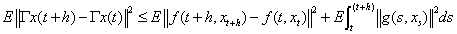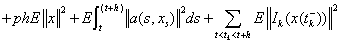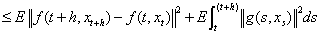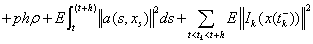as h→ 0, the right hand side of the above inequality tends to zero. This implies the equicontinuity on J \ {t1, t2, ....tm}.

It remains to examine at t=ti, i=1, 2, ....m. Let h ≠ 0 be such that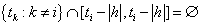Thus we have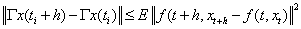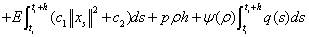The right hand side of the above inequality tends to zero as h → 0. The equicontinuity on J0 follows from the uniform continuity of φ on this interval.

Step 3: Now we show that Γ is continuous

Let {xn}⊂ Ω be a sequence such that xn → x. We will show that Γxn → Γx.

For t ∈ J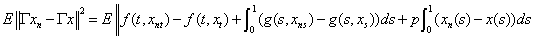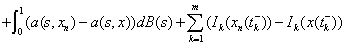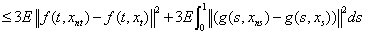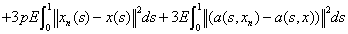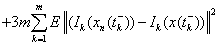Using H3 it can be easily shown that the function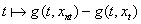is Lebesgue integrable. By the continuity of f and Ik, k=1, 2, ...m and the dominated convergence theorem, the right hand side of inequality (3.2) tends to zero as n → ∞, which completes the proof that Γ is continuous [15-18].

As a sequence of steps 1 to 3, together with the Arzela-Ascoli theorem, we conclude that Γ is completely continuous.

To complete the proof of the theorem, it suffices to prove the following step.

Step 4:

There exists a priori bound of the set

ς={x ∈ Ω: λx=Γx for some λ>1}

Let x ∈ ς and λ>1 be such that λx=Γx. Then x|[−r, t1] satisfies for each t ∈ [0, t1],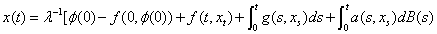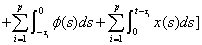It is easy to verify that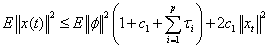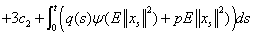(3.3)

Consider the function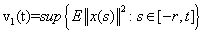, for t ∈ [0, t1]. We have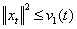for all t ∈ [0, t1] and there is a point t* ∈ [−r, t] such that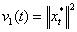If t* < 0, we have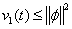for all t ∈ [0, t1]. Now, if t* ≥ 0 from (3.3) it follows that,

for t ∈ [0, t1],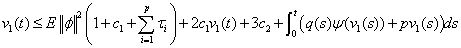And hence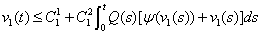Where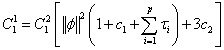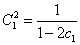Set,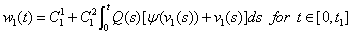Then we have v1(t) ≤ w1(t) for all t ∈ [0, t1]

A direct differentiation of w1 yields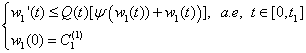By integration, this gives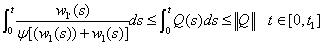(3.4)

By a change of variables, inequality (3.4) becomes,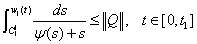By (3.1) and the mean value theorem, there is a constant M1=M1(t1)>0 such that w1(t) ≤ M1 for all t ∈ [0, t1]

That is v1(t) ≤ M1 for all t ∈ [0, t1]

At last we choose M1 such that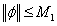to get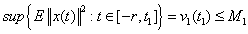Now, consider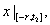satisfies for each t ∈ [0, t2]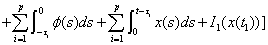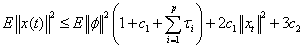Denote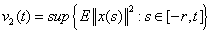for t ∈ [0, t2], Then for each t ∈ [0, t2], we have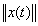, such that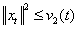.

Let t* ∈ [−r, t] be such that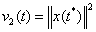.

If t* < 0, we have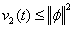for all t ∈ [0, t2].

Now if t*≥ 0, then by (3.5) we have for t ∈ [0, t2]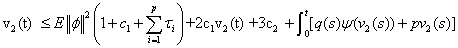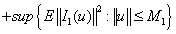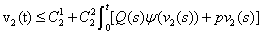Where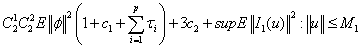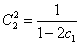And Q(t)=Max {q(t), p} , for t ∈ [0, t2].

If we set,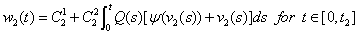for t ∈ [0, t2]

Then we get v2(t) ≤ w2(t) for all t ∈ [0, t2] and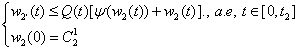These yields,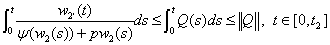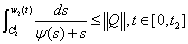Again by (3.1) and the mean value theorem, there is a constant M2=M2 (t1, t2)>0 such that w2(t) ≤ M2 for all t ∈ [0, t2 ], and then v2(t) ≤ M2 for all t ∈ [0, t2]. Finally, if we

Choose M2 such that φ ≤ M2, we get,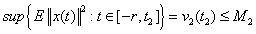Continue this process for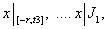we obtain that there exists a constant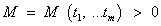such that x ≤ M.

This finish to show that the ζ is bounded in Ω.

As a result the conclusion of theorem holds and consequently the problem (1.1) has a solution x on J1. This completes the proof .

#### References

Select your language of interest to view the total content in your interested language

### Article Usage

• Total views: 12701
• [From(publication date):
November-2015 - Nov 22, 2019]
• Breakdown by view type
• HTML page views : 8716Can't read the image? click here to refresh